# FocalLoss with multi class

I’d like to experiment with using FocalLoss on an imbalanced multiclass problem. Here the activation function would be softmax rather than sigmoid. As a first step I have implemented the following as a test to simply replace the standard cross entropy loss. I expected it to produce the same or similar results to the default but the behavior is different…

``````def one_hot_embedding(labels, num_classes):

class myCCELoss(nn.Module):

def __init__(self):
super(myCCELoss, self).__init__()

def forward(self, input, target):
y = one_hot_embedding(target, input.size(-1))
logit = F.softmax(input)

loss = -1 * V(y) * torch.log(logit) # cross entropy loss
return loss.sum()

learn.crit = myCCELoss
``````

Applying this to a multiclass problem by setting learn.crit = myCCELoss I expected to get the same or similar behavior… I indeed get similar results during training but there are two major differences
1a) the calculated loss is much higher than normal
1b) the learning rate has to be much lower
I get very similar results to using the default loss function but…
2) when unfreezing the net to train all layers the math appears to blow up and fail.

1: Here is the output using the default loss function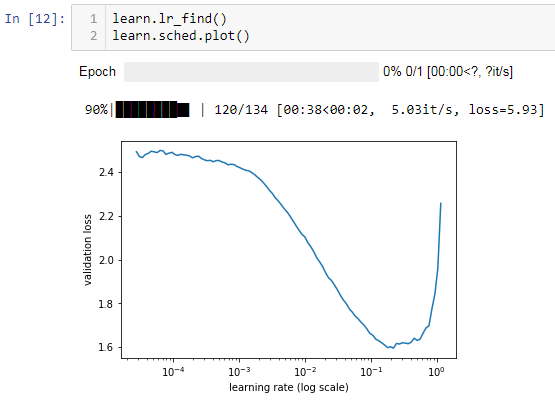and here when restarting but using myCCELoss instead: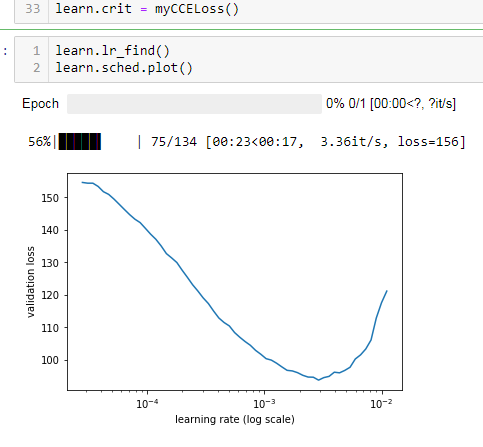Note how much higher the reported loss is and how much lower I have to set the learning rate… (i used 0.002 vs 0.01)

In both cases I train on the same single fold of my data and end up with very similar accuracy and confusion results but the losses during training are always much higher.
here is trainig using the default loss function: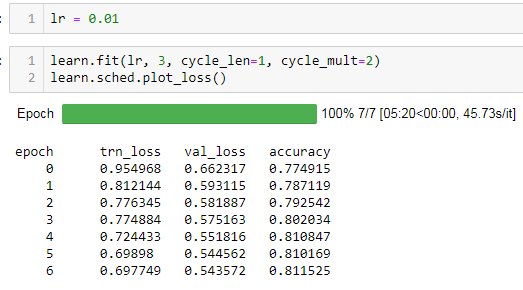and here using myCCELoss: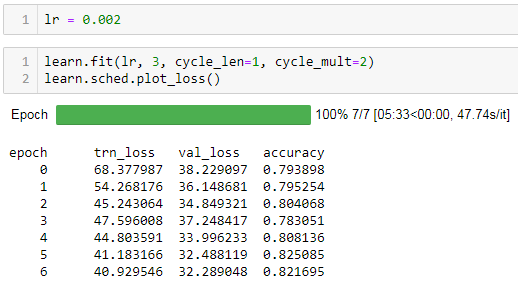Given that the accuracies and confusion matricies are very similar the loss function appears to be working. however when i unfreeze the model (exact same code in both cases) the regular code version works as expected but the one using myCCELoss fails with nans.

Here is the output from default loss function: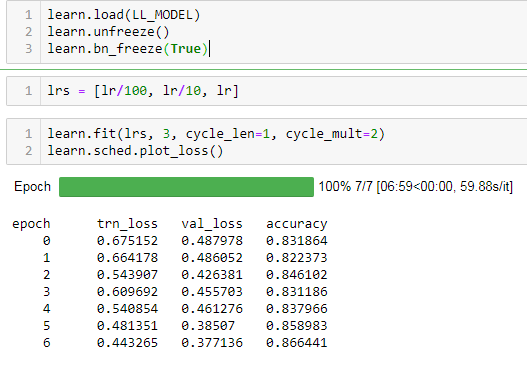and again using myCCELoss: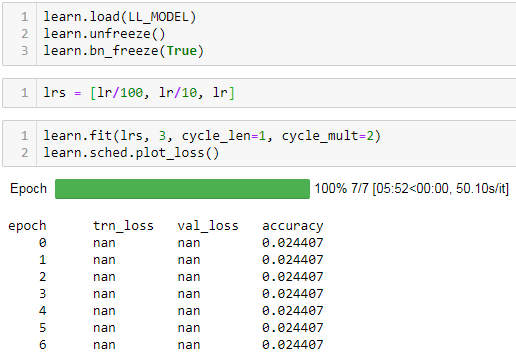What is wrong with myCCELoss? What am I missing?

Thanks for any help!

note for FocalLoss I should just need to scale the loss by:
loss = loss * (1 - logit) ** gamma

1 Like

Note that by reducing my learning rate by an additional power of 10, i.e. lr = 0.0002 I was able to get through the training the unfrozen network with myCCELoss to similar accuracy as the default.

still puzzled as to why the loss values are so high and learning rate has to be low

I found my error. In mySSELoss I was summing the losses over the minibatch rather than averaging them.
in https://pytorch.org/docs/master/nn.html#crossentropyloss
it says: The losses are averaged across observations for each minibatch.
Here is the corrected code

``````def one_hot_embedding(labels, num_classes):

class myCCELoss(nn.Module):

def __init__(self):
super(myCCELoss, self).__init__()

def forward(self, input, target):
y = one_hot_embedding(target, input.size(-1))
logit = F.softmax(input)

loss = -1 * V(y) * torch.log(logit) # cross entropy loss
return loss.sum(dim=1).mean()

class FocalLoss(nn.Module):

def __init__(self, gamma=0, eps=1e-7):
super(FocalLoss, self).__init__()
self.gamma = gamma
self.eps = eps

def forward(self, input, target):
y = one_hot_embedding(target, input.size(-1))
logit = F.softmax(input)
logit = logit.clamp(self.eps, 1. - self.eps)

loss = -1 * V(y) * torch.log(logit) # cross entropy
loss = loss * (1 - logit) ** self.gamma # focal loss
return loss.sum(dim=1).mean()

learn.crit = FocalLoss(gamma=2.0)
``````
4 Likes